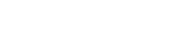# math.atan

`FLOATmath.atanFLOATx`

Available inall subroutines.

Computes the principal value of the arc tangent of its argument `x`.

## Parameters

`x` - Floating point value.

## Return Value

Upon successful completion, this function returns the arc tangent of `x` in the range -`math.PI_2` to `math.PI_2` radians inclusive.

If `x` is `math.NAN`, a NaN will be returned.

If `x` is ±0, `x` will be returned.

If `x` is `math.POS_INFINITY` or `math.NEG_INFINITY`, ±`math.PI_2` will be returned.

If `x` is subnormal, a range error occurs and `x` will be returned.

## Errors

If the `x` argument is subnormal, then fastly.error will be set to `ERANGE`.

## Example

``````declare local var.fo FLOAT;
set var.fo = math.atan(1);``````

BETA